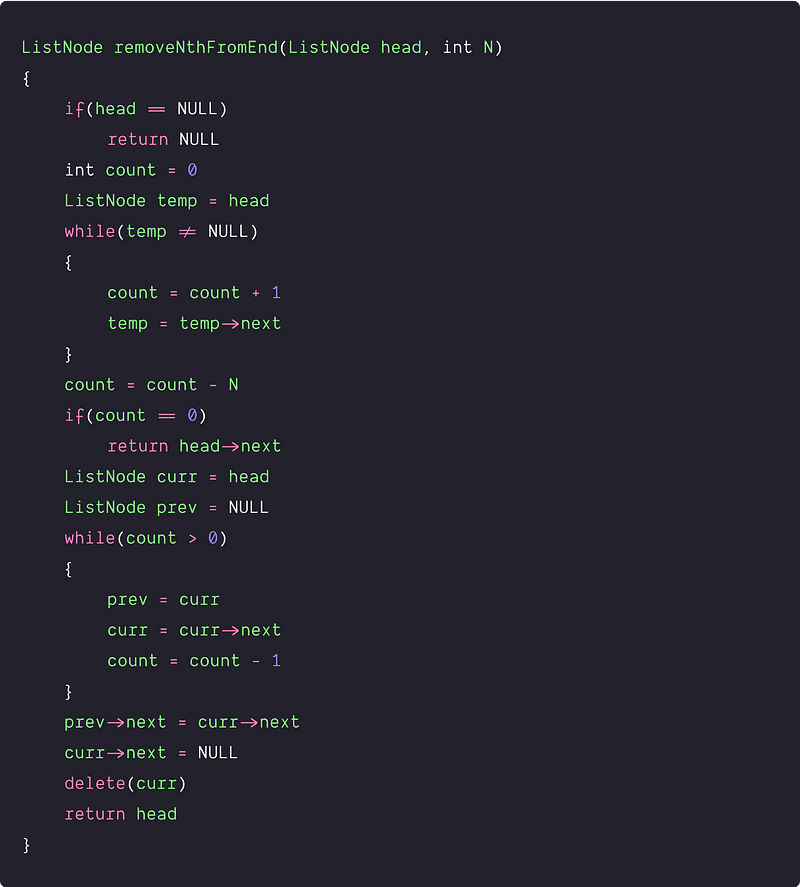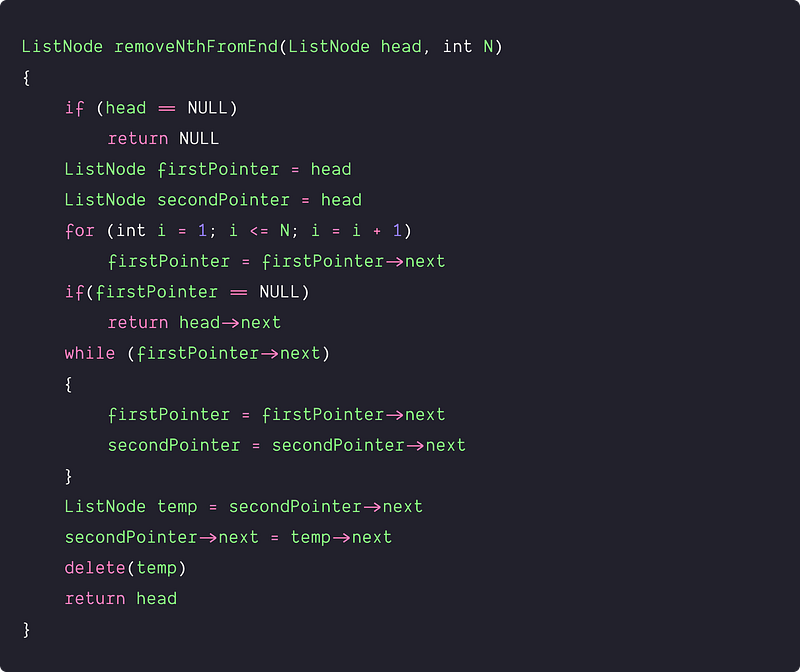# Remove Nth Node from List End

Key takeaway: A good problem for beginners to learn problem-solving in the linked list. This blog will guide you to work efficiently with a linked list and deal with pointers.

### Let’s understand the problem

Write a program to remove the Nth node from the end of the linked list i.e. when the node is traversed from the end, delete the Nth node from there.

Examples

Input : 1->2->3->4->5 , N = 3
Output : 1->2->4->5

Input : 7->8->4->5->3 , N = 1
Output : 7->8->4->5

### Discussed solution approaches

• An iterative approach using double scan
• An iterative approach using two pointers

### An iterative approach using double scan

Solution Idea

Suppose M is the length of the linked list, so one important thing to notice here is that the Nth node from the end is the (M - N + 1)th node from the beginning. Hence the problem could be modified to remove the (M − N + 1)th node from the beginning of the list.

Solution Steps

• Traverse the list and find the length M.
• Again traverse the linked list from the start till we reach the (M − N)th node.
• Now we delete the (M - N + 1)th node and return the head pointer. Before deleting the node, we need to relink the next pointer of the (M - N)th node to the (M − N + 2)th node.

Solution PseudocodeTime and space complexity analysis

We are doing two traversals of the linked list: first to calculate list length M and second to find the (M − N)th node. There are 2M − N operations, so time complexity in the worst-case = O(M). Space complexity = O(1), as constant space is used here.

### An iterative approach using two pointers

Solution Idea

The idea here is to use two pointers and find the (N + 1)th node from the end. Suppose in the beginning, the first pointer is at the (N + 1)th node from the start, and the second pointer is at the head of the linked list. Now, if we move both pointers one step at a time till the first pointer reaches the end, what will happen? The second pointer will point to the (N + 1) node from the end, and we can easily remove the next node i.e. the Nth node from the end.

Solution Steps

• We initialize two pointers: firstPointer and secondPointer. Here secondPointer is at the first node of the linked list, and firstPointer is at (N + 1)th node from the beginning.
• Now, both pointers are exactly separated by N nodes apart. We maintain this constant gap and advance both pointers together until the firstPointer reaches the last node.
• Now, the secondPointer will be pointing to the (N + 1)th node from the end. So, we can easily remove the Nth node from the end by relinking the next pointer of the secondPointer to the next pointer of the next node!

Solution PseudocodeTime and space complexity analysis

If the length of the linked list is M, then the firstPointer will move M steps, and the secondPointer will move M - N steps. So pointer movement = 2M - N and time complexity = O(M). Space complexity = O(1), as we are using constant space.

### Critical ideas to think!

• What would be the best and worst-case scenarios of both approaches?
• Both algorithms take O(M) time, but which one performs fewer operations in the worst-case scenario?
• Sometimes, a "dummy" node is used to simplify some corner cases, such as a list with only one node or removing the head of the list. In other words, having dummy nodes in any kind of linked list, singly, double or circular, makes the corner cased vanish. Thus, programming becomes a lot easier. Now critical question is: how do we use the idea of dummy node in the above approaches to handle the corner cases?
• In the 2nd approach, we use two loops: one for taking firstPointer to the (N + 1)th node and the other for finding the (N+1)th node from the end. Can we merge these two processes and implement them using a single loop?
• Can we try to solve this problem using recursion?
• Can we implement the two-pointers solution differently?

### Comparison of time and space complexities

• An iterative approach using double scan: Time = O(M), Space = O(1)
• An iterative approach using two pointers: Time = O(M), Space = O(1)

### Suggested problems to practice

Enjoy learning, Enjoy algorithms!

Get well-designed application and interview centirc content on ds-algorithms, machine learning, system design and oops. Content will be delivered weekly.